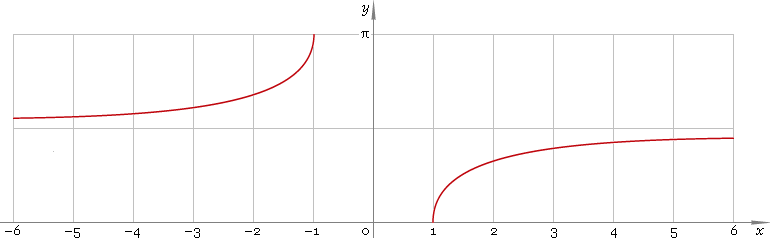The Art of Mathematics

# arcsec — trigonometric arc secant function

## 1. Definition

Arc secant is inverse of the secant function.

arcsecxsecinvx

## 2. Graph

Arc secant is discontinuous function defined on all real axis except the (−1, 1) range — so its domain is (−∞, −1]∪[1, +∞). Function graph is depicted below — fig. 1.Fig. 1. Graph of the arc secant function y = arcsecx.

Function codomain is limited to the range [0, π/2)∪(π/2, π].

## 3. Identities

Complementary angle:

arcsecx + arccscx = π/2

and as consequence:

arcsec csc φ = π/2 − φ

Negative argument:

arcsec(−x) = π − arcsecx

Reciprocal argument:

arsec(1/x) = arccosx

Sum and difference:

arcsecx + arcsecy = arcsec(xy /{1 − xy√[(1 − 1 /x2)(1 − 1 /y2)]})
arcsecx − arcsecy = arcsec(xy /{1 + xy√[(1 − 1 /x2)(1 − 1 /y2)]})

Some argument values:

Argument xValue arcsecx
10
√6 − √2π/12
√(50 − 10√5) /5π/10
√(2 − √2)π/8
2√3 /3π/6
√5 − 1π/5
√2π/4
√(50 + 10√5) /53π/10
2π/3
√(4 + 2√2)3π/8
√5 + 12π/5
√6 + √25π/12
Table 1. Arc secant for some argument values.

## 4. Derivative and indefinite integral

Arc secant derivative:

arcsec′x = 1 /[|x| √(x2 − 1)]

Indefinite integral of the arc secant:

∫ arcsecx dx = x arcsecx − signx ln|x + √(x2 − 1)| + C = x arcsecx − arcosh|x| + C

where sign is a signum function and C is an arbitrary constant.

## 5. How to use

To calculate arc secant of the number:

``arcsec(−1);``

To get arc secant of the complex number:

``arcsec(−1−i);``

To get arc secant of the current result:

``arcsec(rslt);``

To get arc secant of the number z in calculator memory:

``arcsec(mem[z]);``

## 6. Support

Trigonometric arc secant of the real argument is supported in free version of the Librow calculator.

Trigonometric arc secant of the complex argument is supported in professional version of the Librow calculator.